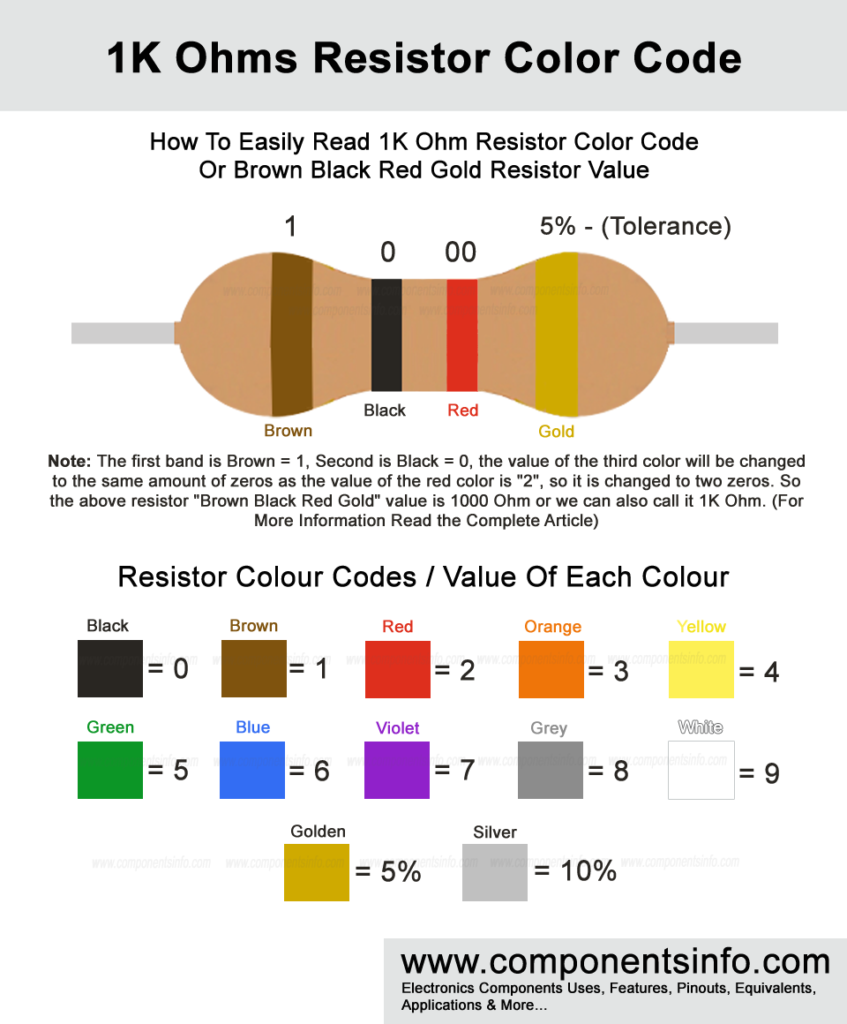# 1K Ohm Resistor Color Code Or Brown Black Red Gold Resistor Value

If you are looking for 1K ohm resistor color code or brown black red gold resistor value then you have landed at the right place. This page contains all the important information about how to easily read 1K resistor codes or brown black red gold resistor value.

Resistors are passive electronic components designed to limit the current in electronic circuits. Current limiting is very common in electronic circuits to provide only the required current to electronic components so they can work properly. For example, to safely drive an LED we always require a suitable value resistor according to the voltage applied. Moreover, to control a transistor properly we have to provide the required current at the base of the transistor in that case we also have to use a required resistor at the base of the transistor, these are just a few examples of how resistors are used in electronic circuits and in different situation we have to use different values of resistor required by the circuit or component to limit the current to a specific level, therefore, there are wide range of fixed value resistors are available that we can use in our circuits but if you can’t find a required value of a resistor then you can easily make it by yourself. We will guide you on how you can do that easily.#### Reading 1K Ohm Resistor Color Code Or Brown Black Red Gold Resistor Value

Reading resistor values are not very difficult and one can easily read them by checking or learning the value or code of each color printed on the resistor’s body. There is a standard color code chart that is developed by (RMS) Radio Manufacturers Association in 1920s from that time all resistor manufacturers follow these standard color codes to print them on the resistor to show their value. Therefore if you want to know the value of any resistor and its tolerance then you have to check this color code chart. In the image above we can see a resistor color code chart and also a 1K resistor. To read the value of this resistor first we have to check the value of the first color code which is brown and its value is “1”, then we check the value of the second color band which is black and its value is “0”, reading the third color band is a bit different. Any color that is present on the third number its value will be converted to the same amount of zeros. Such as the value or color code of red is “2” so we will use two zeros which has also shown in the image above. In the end, we will combine these three values and our resistor value will be known which will be: 1000 Ohms or 1K Ohms.

There is also another band which is the fourth band which is golden in color. This band should be ignored when calculating the resistor value. This band shows the tolerance of a resistor means how much the value of the resistor varies from its actual value when the temperature changes so if the fourth color is golden it means that the resistor has 5% tolerance means the actual value of the resistor will be 5% vary. Sometimes the fourth band is silver which means the resistor has a 10% tolerance.

#### Making a Quick Resistor When a Fixed Value Resistor is Not Available

It is important to have some standard values of fixed value resistors in your lab or table as well as some variable resistor values because sometimes we require some resistor values which are not very common so in this case we can make that value with a variable resistor which will save a lot of time to go to the market and buying that resistor value or order online.